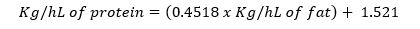# Calculations

The following steps are required to calculate the amount of powder or skim milk to be added, or the amount of cream to be removed. Suppose a cheese maker wishes to fill a 10,000 l (100 hl) setting vat for the manufacture of Cheddar cheese.

Step 1. Determine the protein and fat contents of the milk using an automatic milk analyzer. If a milk analyser is not available the protein content of pooled milk can be crudely estimated from the fat content using the following formula:

Kg/hL of protein = (0.4518 x Kg/hL of fat) + l.521For the purpose of this example, assume the available milk contains 3.50 Kg/hL of fat and 3.l0 Kg/hL of protein.

Step 2. Determine the required fat, moisture and F/DM of Cheddar cheese. 'Dairy Products Regulations' of the Canada Agricultural Products Standards Act require Cheddar cheese to contain a minimum of 31% fat and a maximum of 39% moisture. Therefore,

FDM = % fat/% dry matter = 30.0/(100.0 - 39.0) = 49.2%Step 3. Determine the required P/F of the milk. The P/F required to yield F/DM = 50% as required for Cheddar cheese is about 0.96.

Step 4. Calculate the amount of: skim milk powder to be added; or fat to be removed; or skim milk to be added.

Standardization by Adding skim milk powder

(i) Calculate the % protein required to give P/F = 0.96

The required level of protein = 0.96 x % fat = 0.96 x 3.50 = 3.36

(ii) The % protein to be added = 3.36 - 3.10 = 0.26 Kg/hL

(iii) Calculate the weight of protein which must be added per 100.00 hL of milk.

The required weight of protein = 0.26 Kg/hL x 100 hL = 26.0 Kg

(iv) Calculate the amount of powder which must be added assuming the skim milk powder (SMP) contains 35.0% protein. If possible the skim powder should be analyzed so the exact protein content is known. The supplier may be able to provide this information. Protein content can also be estimated using a milk analyzer to test the reconstituted skim milk.

Required amount of powder = 26.0 Kg/0.35 = 74.3 Kg

(v) Check calculations:

Weight of fat in milk: 3.50 Kg/hL x 100.00 hL = 350.0 Kg.
Weight of protein in milk: 3.10 Kg/hL x 100.00 hL = 310.0 Kg
Weight of protein in SMP: 0.35 Kg/Kg x 74.0 Kg = 26.0 Kg
Total Protein: 310.0 Kg + 26.0 Kg = 336.0 Kg
P/F ratio of standardized milk: 336.0 Kg/350.0 Kg = 0.96

Standardization by Removing fat

(i) Calculate the level of fat required to give P/F = 0.96.

The required level of fat = Kg/hL of protein/.96 = 3.10 Kg/hL/.96 = 3.23 Kg/hL

(ii) Use a Pearson's square to calculate the litres of cream that must be removed, assuming that the separator removes cream containing 30.00 kg/hl of fat.

 Un-standardized Milk 3.50 Kg/hL 30.00 - 3.23 = 26.77 Parts Stanrdardized Milk Standardized Milk 3.23 Kg/hl Cream 30.00 Kg/hL 3.50 - 3.23 = 0.27 Parts Cream Total Parts 26.77 + 0.27 = 27.04

This means that the required proportions of cream and fresh milk are 0.27 and 26.77 parts, respectively, for a total of 0.27 + 26.77 = 27.04 parts. On a percent basis, the components are:

Standardized Milk 100 x 26.77/27.04 = 99.0% w/v 100 x 0.27/27.04 = 1.00% w/v

(iii) Calculate how much cream must be removed from 10,000 Kg of milk to provide standardized milk containing 3.23% fat.

Cream to be removed = 1.00% of 100 hL = 1.00 hL.

(iv) Check calculations:

Weight of fat in milk 3.50 Kg/hL x 100.00 hL = 350.0 Kg 30.00 Kg/hL x 1.00 hL = 30.0 Kg 350.0 Kg - 30.0 Kg = 320.0 Kg 100.00 hL - 1.00 hL = 99.0 hL 3.10 Kg/hL x 99.0 hL = 306.9 Kg 306.9/320.0 = 0.960

(v) Adjust the final weight for the quantity of cream removed. If you wish to fill the vat completely sum the vat capacity and the initial estimate of the cream to be removed and recalculate the required amount of cream.

Approximate total volume of fresh milk: 100.00 hL + 1.01 hL = 101.01 hL. 1.00% of 101.01 hL = 1.01 hL 100.00 hL - 1.01 hL = 99.99 hL

The following calculation is based on the assumption that the protein content of the skim milk is the same as the protein content in the skim portion of the fresh milk to be standardized. This is exactly true only when the skim milk is derived from the same source as the fresh milk.

(i) Use a Pearson square to determine the relative proportions of skim milk and milk required to yield a fat content of 3.23% as calculated in Step B above.

 Skim milk 0.10 Kg/hL 3.5 - 3.23 = 0.27 Parts Skim Milk Standardized Milk 3.23 Kg/hl Unstandardized Milk 3.50 Kg/hL 3.23 - 0.10 = 3.13 Parts Unstandardized Milk Total Parts 0.27 + 3.13 = 3.40

This means that 0.27 parts of skim are required for 3.13 parts of milk where the total mixture consists of 0.27 + 3.13 = 3.40 parts. On a percent basis, the mixture is:

Skim milk 100 x 0.27/3.4 = 7.9% 100 x 3.13/3.4 = 92.1%

(ii) Calculate the amount of skim and fresh milk required.

Weight unstandardized milk 92.1% of 100 hL = 92.10 hL. 7.9% of 100 hL = 7.90 hL.

(iii) Check:

Weight of fat in unstandardized milk 3.50 Kg/hL x 92.10 hL = 322.4 Kg 0.10 Kg/hL x 7.90 hL = 0.80 Kg 322.4 Kg + 0.8 Kg = 323.2 Kg 3.10 Kg/hL x 100 hL = 310.0 Kg. 310.0/323.2 = 0.959